#### 期刊菜单

Research on the Operation Strategy of Enterprise User-Generated Content (UGC) Based on Information Asymmetry

Abstract: Under word-of-mouth driven consumer behavior, firms invest a sum of money in operations to manage consumer-generated content (UGC) interventions to stimulate more consumers to purchase products in order to leverage consumer recommendations. In this context, this paper constructs a two-stage strategic competition game model between firms and consumers under the asymmetry of firm quality information to investigate whether new consumer brand firms should pay information rent to achieve signaling through UGC operational input decisions so as to disclose their true type to consumers, and this paper also investigates the impact of consumers’ sensitivity to user-generated content (UGC) on firms’ decisions. It is found that: under complete information, enterprises with high quality types are more willing to invest high cost in UGC operation; under incomplete information, the signaling game between enterprises and consumers can get both separated equilibrium solution and mixed equilibrium solution, and the enterprises’ choice of separated equilibrium or mixed equilibrium is related to the initial probability of enterprises with high types and the quality difference between enterprises with high and low types; meanwhile, this paper beaches for a long time how consumers’ sensitivity to UGC content affects enterprises’ decision. At the same time, this paper studies how consumers’ sensitivity to UGC content affects enterprises’ UGC operation input decisions.

1. 引言

2. 文献综述

3. 模型说明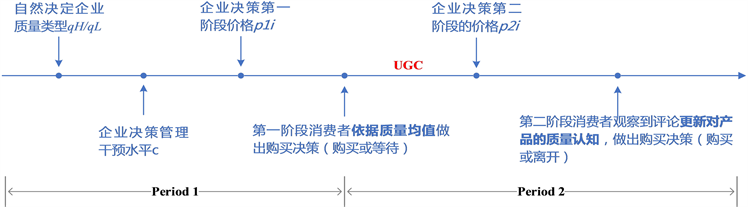Figure 1. Event sequence

4. 完全信息条件下的企业决策

${U}_{2}=v+{q}_{j}-{p}_{2i}+\beta e\ge 0⇔v\ge \underset{_}{{v}_{i}}={p}_{2i}-{q}_{j}-\beta e$

$\underset{{p}_{2i}}{\mathrm{max}}{\pi }_{2i}=\left(\stackrel{¯}{v}-\mathrm{max}\left\{\underset{_}{{v}_{i}},0\right\}\right)\ast \left({p}_{2i}-\gamma {q}_{i}\right)$ (1)

$\stackrel{¯}{{v}_{i}}=\text{min}\left\{v\in \left[0,1\right]:v+\mu -{p}_{1i}\ge v+{q}_{j}-{p}_{2i}+\beta e\right\}$

$\underset{{p}_{1i},{e}_{i}}{\mathrm{max}}{\pi }_{1i}=\left(1-\stackrel{¯}{{v}_{i}}\right)*\left({p}_{1i}-\gamma {q}_{i}\right)-\frac{k{e}_{i}^{2}}{2}$ (2)

(1) 企业第二阶段的最优定价策略为：

${\stackrel{¯}{p}}_{2i}^{*}=\frac{{\beta }^{2}\left(1+{q}_{i}-{q}_{i}\gamma \right)}{2k-5{\beta }^{2}}+\frac{k\left(1+{q}_{i}+{q}_{i}\gamma \right)-{\beta }^{2}\left(2+{q}_{i}\left(2+3\gamma \right)\right)}{2k-5{\beta }^{2}}$，对应的收益为 ${\stackrel{¯}{\pi }}_{2i}^{*}=\frac{{\left(k-{\beta }^{2}\right)}^{2}{\left(-1+{q}_{i}\left(-1+\gamma \right)\right)}^{2}}{{\left(2k-5{\beta }^{2}\right)}^{2}}$$i\in \left\{H,L\right\}$

(2) 企业第一阶段的最优策略为 $\left\{{\stackrel{¯}{p}}_{1i}^{*},{\stackrel{¯}{e}}_{i}^{*}\right\}$，其中 ${\stackrel{¯}{p}}_{1i}^{*}=\frac{k\left(1+{q}_{i}\left(1+\gamma \right)\right)-{\beta }^{2}\left(2+{q}_{i}\left(2+3\gamma \right)\right)}{2k-5{\beta }^{2}}$${\stackrel{¯}{e}}_{i}^{*}=\frac{\beta \left(1+{q}_{i}\left(1-\gamma \right)\right)}{2k-5{\beta }^{2}}$，对应的收益为 ${\stackrel{¯}{\pi }}_{i}^{*}=\frac{\left(k-2{\beta }^{2}\right){\left(-1+{q}_{i}\left(-1+\gamma \right)\right)}^{2}}{4k-10{\beta }^{2}}$$i\in \left\{H,L\right\}$

5. 不完全信息条件下的企业决策

5.1. 企业模仿动机分析$\left(a=0.8,\beta =0.38,{q}_{H}=2.25,{q}_{L}=2.19,\gamma =0.28,k=0.73\right)$

Figure 2. Potential corporate revenue

5.2. 分离均衡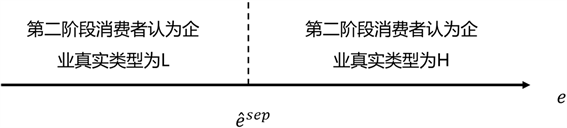Figure 3. Belief structure of consumers in the separation equilibrium

${q}_{j}=\left\{\begin{array}{c}{q}_{H},e>{\stackrel{^}{e}}^{sep}\\ {q}_{L},e\le {\stackrel{^}{e}}^{sep}\end{array}$

${\pi }_{LH}\left({e}_{H}^{sep*}\right)\le {\pi }_{LL}^{*}\left({e}_{L}^{sep*}\right)$

$\theta >\frac{4\left(-k-5kqH+4k\left(qL\left(1-\alpha \right)+qH\alpha \right)+kqH\gamma \right)}{-10k+4k\gamma -{\beta }^{2}{\gamma }^{2}}=\stackrel{¯}{\theta }$.

$\underset{{\stackrel{^}{e}}^{sep}}{\mathrm{max}}{\pi }_{H}^{sep}\left({\stackrel{^}{e}}_{H}^{sep},{p}_{1i}\right)={d}_{1}*\left({p}_{1H}-\gamma {q}_{H}\right)-\frac{k{\stackrel{^}{e}}_{H}^{sep}{}^{2}}{2}$

(1) 低质量企业UGC运营投入 ${e}_{L}^{sep*}={e}_{L}^{*}$${\pi }_{L}^{sep}={\stackrel{¯}{\pi }}_{L}^{*}$

(2) 高质量企业UGC运营投入 ${e}_{H}^{sep*}=\left\{\begin{array}{l}{e}_{H}^{*}，\theta >\stackrel{¯}{\theta }\\ {e}_{H}^{sep*},\theta \le \stackrel{¯}{\theta }\end{array}$，其中

${e}_{H}^{sep*}=\frac{\beta +qH\left(5-4\alpha \right)\beta +qL\beta \left(-4+4\alpha -\gamma \right)+\sqrt{2}\sqrt{-k\left(qH-qL\right)\left(-2+qH\left(-5+8\alpha \right)+qL\left(3-8\alpha +2\gamma \right)\right)}}{2k-5{\beta }^{2}}$

(3) 高类型企业UGC运营投入扭曲的幅度 $\Delta e={e}_{H}^{sep*}-{e}_{H}^{*}>0$$\Delta w={\stackrel{¯}{w}}_{H}^{sig*}-{w}^{sd*}$ 随着消费者对在线UGC内容的敏感程度 $\beta$ 增大而增大。

(1) i类型企业第二阶段最优策略 ${p}_{2i}^{sep*}=\frac{k\left(1+3{q}_{i}+{q}_{i}\gamma -2\mu \right)-{\beta }^{2}\left(1+4{q}_{i}\gamma +\mu \right)}{2k-5{\beta }^{2}}$

(2) H企业第一阶段最优定价策略 ${p}_{1H}^{sep*}=\left\{\begin{array}{l}\frac{1}{2}\left(1+{q}_{H}+{q}_{H}\gamma +\frac{{\beta }^{2}\left(1+5{q}_{H}-{q}_{H}\gamma -4\mu \right)}{2k-5{\beta }^{2}}\right),\text{if}\theta \ge \stackrel{¯}{\theta }\\ \frac{\beta \left(1+5{q}_{H}-{q}_{H}\gamma -4\mu \right)}{2k-5{\beta }^{2}},\text{if}\theta <\stackrel{¯}{\theta }\end{array}$，最优UGC运营投放策略为 ${e}_{H}^{sep*}=\left\{\begin{array}{l}{e}_{H}^{*},\theta >\stackrel{¯}{\theta }\\ {e}_{H}^{sep*},\theta \le \stackrel{¯}{\theta }\end{array}$

$\theta >\stackrel{¯}{\theta }$ 时，两种类型企业间的质量差异很大，低类型企业的最优UGC运营投入与高类型企业的最优UGC运营投入差距很大，换句话说，其在社交媒体上用户生成内容水平差距很大，高类型拥有较好的市场反馈(得益于其前期高运营投入)，而低类型相对来说没有太多消费者在社交媒体上发布关于其产品正向的反馈或评价，因此低类型企业模仿高类型企业的难度很大。低类型企业的模仿行为需要大幅提高自己的UGC运营投入，导致伪装成本增加。此时，尽管低类型企业模仿高类型企业可以获得更高的销售量，但是模仿高类型企业的最优UGC运营投入会导致低类型企业整体收益下降，模仿高类型企业是无利可图的。所以当 $\theta >\stackrel{¯}{\theta }$ 时，高类型企业不需要对UGC运营投入进行扭曲，两类企业自然分离。

$\theta \le \stackrel{¯}{\theta }$ 时，两种类型企业间的质量差距较小，低类型企业模仿高类型企业的难度很小，且低类型企业模仿高类型企业可以获得更高的销售量，从而获得更高的收益。所以当 $\theta \le \stackrel{¯}{\theta }$ 时，为了实现分离，高类型企业需要将自己的UGC运营投入向下扭曲到低类型企业无法模仿，即令自己的UGC运营投入足够低，以至于低类型企业模仿自己时获得的收益无法高于信息对称时的最高收益。

5.3. 混同均衡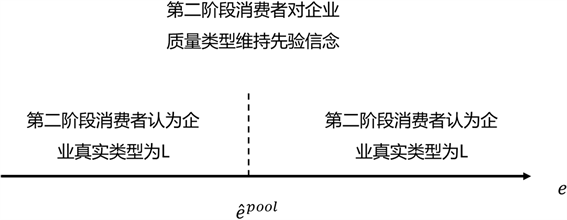Figure 4. Belief structure of consumers in pooling equilibrium

${q}_{j}=\left\{\begin{array}{c}\mu ,e={\stackrel{^}{e}}^{pool}\\ {q}_{L},e\ne {\stackrel{^}{e}}^{pool}\end{array}$

$\underset{{\stackrel{^}{e}}^{pool}}{\mathrm{max}}{\pi }_{H}^{pool}\left({\stackrel{^}{e}}^{pool},{p}_{1H}\right)={d}_{1}*\left({p}_{1H}-\gamma {q}_{H}\right)-\frac{k{\stackrel{^}{e}}^{pool}{}^{2}}{2}$

$\underset{{\stackrel{^}{e}}^{pool}}{\mathrm{max}}{\pi }_{L}^{pool}\left({\stackrel{^}{e}}^{pool},{p}_{1L}\right)={d}_{1}*\left({p}_{1L}-\gamma {q}_{L}\right)-\frac{k{\stackrel{^}{e}}^{pool}{}^{2}}{2}$

${U}_{1}=v+\mu -{p}_{1i}$${U}_{2}=v+{q}_{L}-{p}_{2L}+\beta e$

${\pi }_{HN}\left({\stackrel{^}{e}}^{pool}\right)>{\pi }_{HL}^{*}$

${\pi }_{LN}\left({\stackrel{^}{e}}^{pool}\right)>{\pi }_{LL}^{*}$

${\underset{_}{e}}^{p}\le {\stackrel{¯}{e}}^{p}$

$\stackrel{¯}{\stackrel{¯}{\theta }}\le \frac{4k\alpha \left(1+qH-qH\gamma \right)}{2k\alpha \left(2+3\alpha -2\gamma \right)+{\beta }^{2}{\gamma }^{2}}$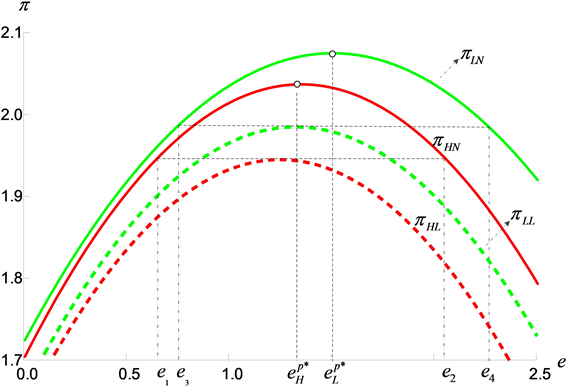$\left(a=0.8,\beta =0.38,{q}_{H}=2.25,{q}_{L}=2.19,\gamma =0.28,k=0.73\right)$

Figure 5. Belief structure of consumers in pooling equilibrium

5.4. 企业均衡策略选择

${\pi }_{H}^{sig}=\mathrm{max}\left\{{\pi }_{H}^{pool*},{\pi }_{H}^{sep*}\right\}$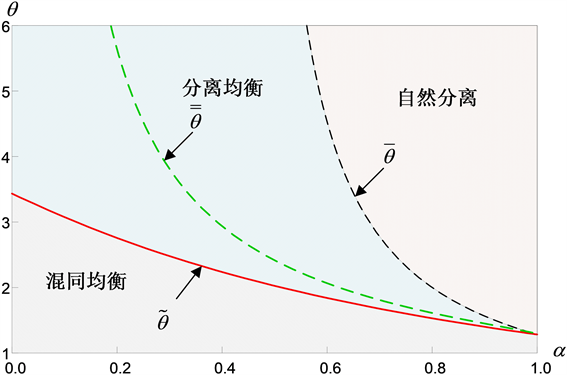$\left(\beta =0.8,{q}_{H}=2.25,\gamma =0.28,k=1.0\right)$

Figure 6. Balanced output of high-type enterprises

5.5. 本章小结

6. 总结与展望

  Saokosal, O. and Han, D.W. (2011) An Empirical Study of the Determinants of the Intention to Participate in User-Created Contents (UCC) Services. Mathematical Proceedings of the Cambridge Philosophical Society, 38, 15110-15121. https://doi.org/10.1016/j.eswa.2011.05.098  杜学美, 丁璟妤, 谢志鸿, 雷丽芳. 在线评论对消费者购买意愿的影响研究[J]. 管理评论, 2016, 28(3): 173-183.  郭恺强, 王洪伟, 郑晗. 基于在线评论的网络零售定价模型研究[J]. 商业经济与管理, 2014(4): 59-66.  Jiang, B.J. and Yang, B.C. (2018) Quality and Pricing Decisions in a Market with Consumer Information Sharing. Management Science, 65, 272-285. https://doi.org/10.1287/mnsc.2017.2930  周建亨, 李颖潇. 顾客忠诚对企业传递成本信号的影响研究[J/OL]. 中国管理科学, 1-12. https://doi.org/10.16381/j.cnki.issn1003-207x.2019.1370, 2021-11-23.  Guo, X. and Jiang, B. (2016) Signaling through Price and Quality to Consumers with Fairness Concerns. Journal of Marketing Research, 53, 988-1000. https://doi.org/10.1509/jmr.15.0323  张琪, 高杰. 竞争市场上保修期与价格的联合质量信号传递作用[J]. 中国管理科学, 2018, 26(7): 71-83.  Ryu, G. and Feick, L. (2007) A Penny for Your Thoughts: Referral Reward Programs and Referral Likelihood. Journal of Marketing, 71, 84-94. https://doi.org/10.1509/jmkg.71.1.084  Kornish, L.J. and Li, Q.P. (2009) Optimal Referral Bonuses with Asymmetric Information: Firm-Offered and Interpersonal Incentives. Marketing Science, 29, 108-121. https://doi.org/10.1287/mksc.1080.0484  Ping, X., Tang, C.S. and Wirtz, J. (2011) Optimizing Referral Reward Programs under Impression Management Considerations. European Journal of Operational Research, 215, 730-739.  朱翊敏, 于洪彦. 奖励额度、分配方案与关系强度对网络推荐意愿的影响[J]. 经济经纬, 2015, 32(5): 120-125.  周丹, 姚忠, 窦一凡. 消费者预算约束对推荐奖励策略的影响研究们. 管理科学学报, 2017, 20(8): 80-92.  Besanko, D. and Winston, W.L. (1990) Optimal Price Skimming by a Monopolist Facing Rational Consumers. Management Science, 36, 555-567. https://doi.org/10.1287/mnsc.36.5.555  Yossi Aviv, A.P. (2008) Optimal Pricing of Seasonal Products in the Presence of Forward-Looking Consumers. Manufacturing Service Operations Management, 10, 339-359. https://doi.org/10.1287/msom.1070.0183  Shum, S., Tong, S.L. and Xiao, T.T. (2016) On the Impact of Uncertain Cost Reduction When Selling to Strategic Customers. Management Science, 63, 843-860. https://doi.org/10.1287/mnsc.2015.2355  Zhang, J., Li, S., Zhang, S., et al. (2019) Manufacturer Encroachment with Quality Decision under Asymmetric Demand Information. European Journal of Operational Research, 273, 217-236. https://doi.org/10.1016/j.ejor.2018.08.002